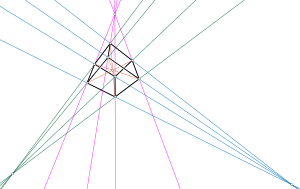# Reye configurationThe Reye configuration

In mathematics, the Reye configuration, introduced by Theodor Reye (1882), is a configuration of 12 points and 16 lines. Each point of the configuration belongs to four lines, and each line contains three points. Therefore, in the notation of configurations, the Reye configuration is written as 124163.

## Realization

The Reye configuration can be realized in three-dimensional projective space by taking the lines to be the 12 edges and four long diagonals of a cube, and the points as the eight vertices of the cube, its center, and the three points where groups of four parallel cube edges meet the plane at infinity. Two regular tetrahedra may be inscribed within a cube, forming a stella octangula; these two tetrahedra are perspective figures to each other in four different ways, and the other four points of the configuration are their centers of perspectivity. These two tetrahedra together with the tetrahedron of the remaining 4 points form a desmic system of three tetrahedra.

Any two disjoint spheres in three dimensional space, with different radii, have two bitangent double cones, the apexes of which are called the centers of similitude. If three spheres are given, with their centers non-collinear, then their six centers of similitude form the six points of a complete quadrilateral, the four lines of which are called the axes of similitude. And if four spheres are given, with their centers non-coplanar, then they determine 12 centers of similitude and 16 axes of similitude, which together form an instance of the Reye configuration (Hilbert & Cohn-Vossen 1952).

The Reye configuration can also be realized by points and lines in the Euclidean plane, by drawing the three-dimensional configuration in three-point perspective. An 83122 configuration of eight points in the real projective plane and 12 lines connecting them, with the connection pattern of a cube, can be extended to form the Reye configuration if and only if the eight points are a perspective projection of a parallelepiped (Servatius & Servatius 2010)

## Application

Aravind (2000) pointed out that the Reye configuration underlies some of the proofs of the Bell–Kochen–Specker theorem about the non-existence of hidden variables in quantum mechanics.

## Related configurations

The Pappus configuration may be formed from two triangles that are perspective figures to each other in three different ways, analogous to the interpretation of the Reye configuration involving desmic tetrahedra.

If the Reye configuration is formed from a cube in three-dimensional space, then there are 12 planes containing four lines each: the six face planes of the cube, and the six planes through pairs of opposite edges of the cube. Intersecting these 12 planes and 16 lines with another plane in general position produces a 163124 configuration, the dual of the Reye configuration. The original Reye configuration and its dual together form a 284284 configuration (Grünbaum & Rigby 1990).

There are 574 distinct configurations of type 124163 (Betten & Betten 2005).